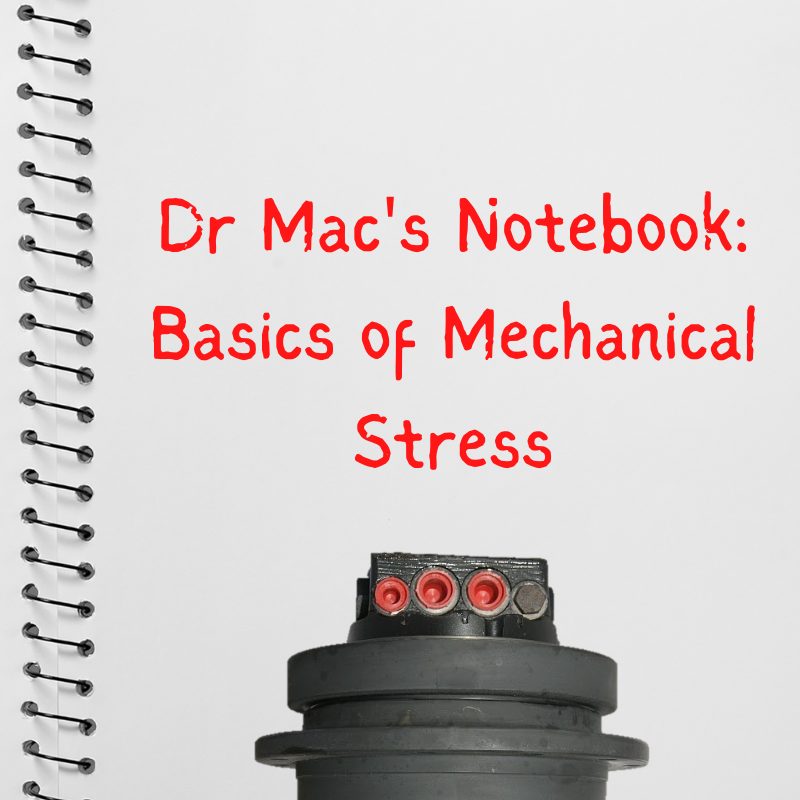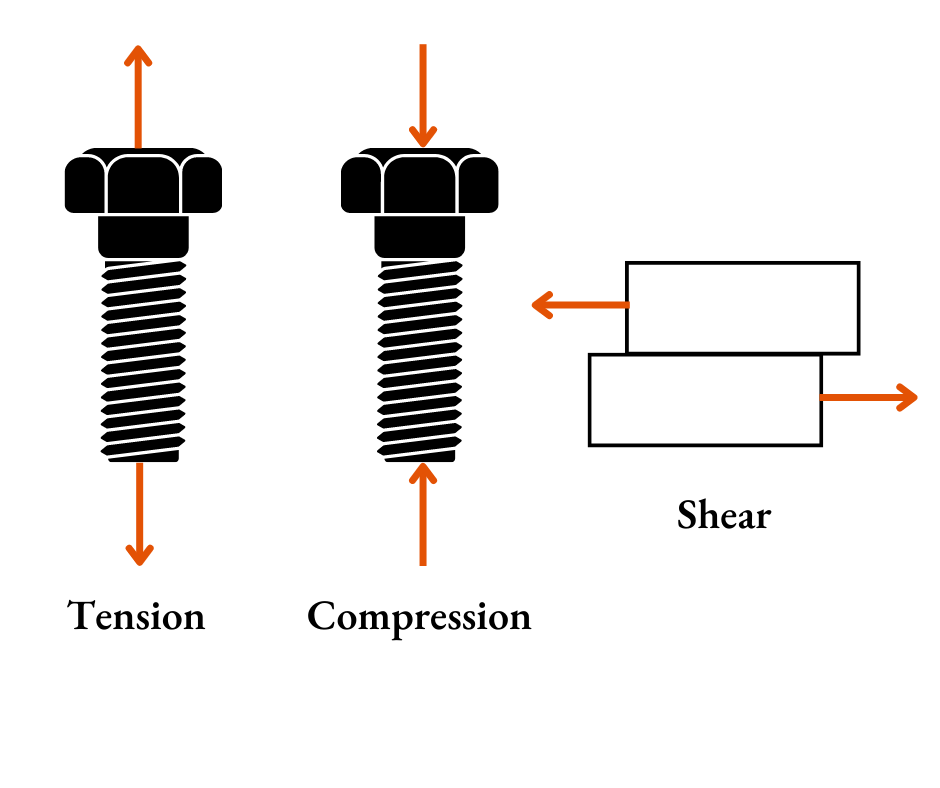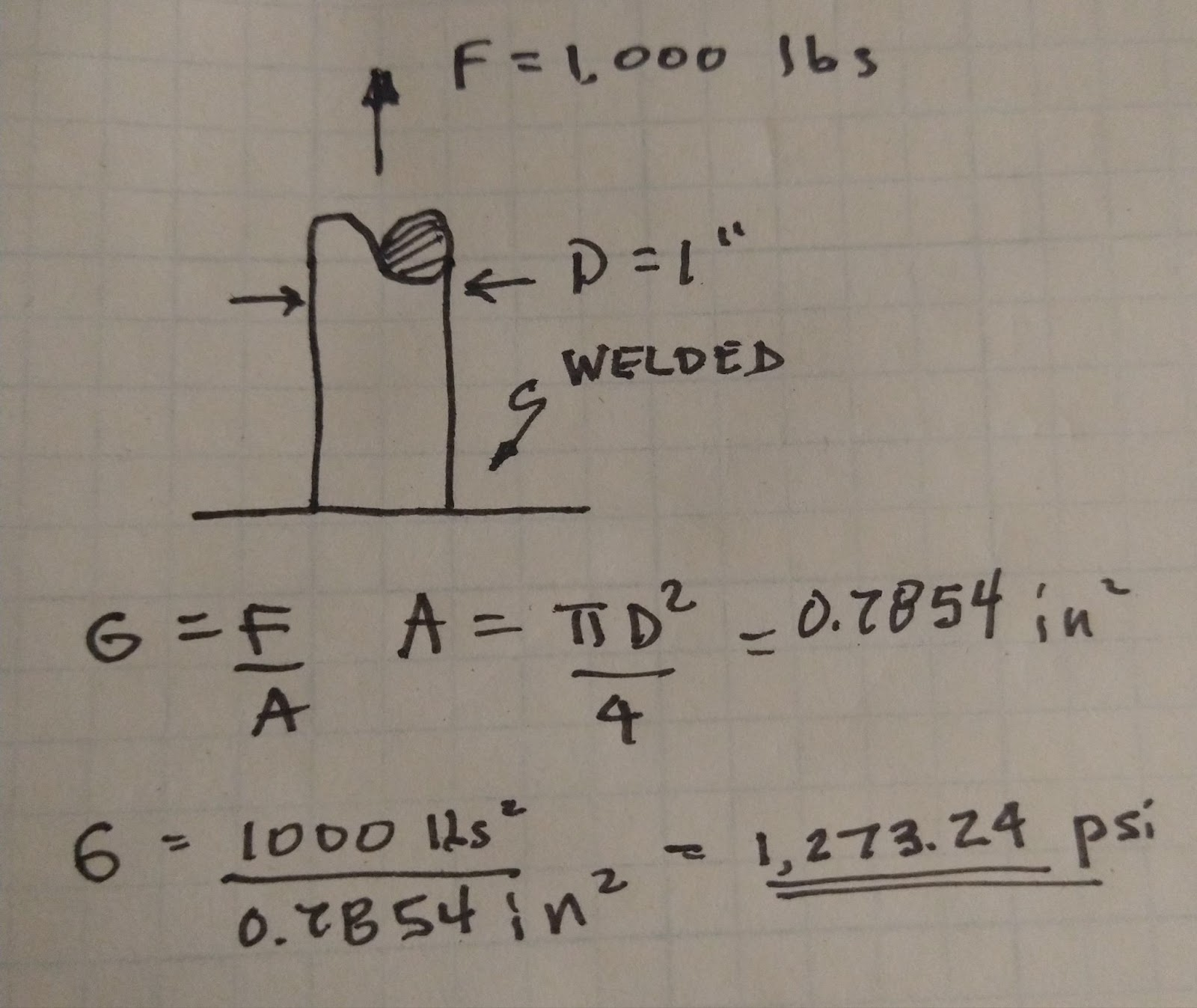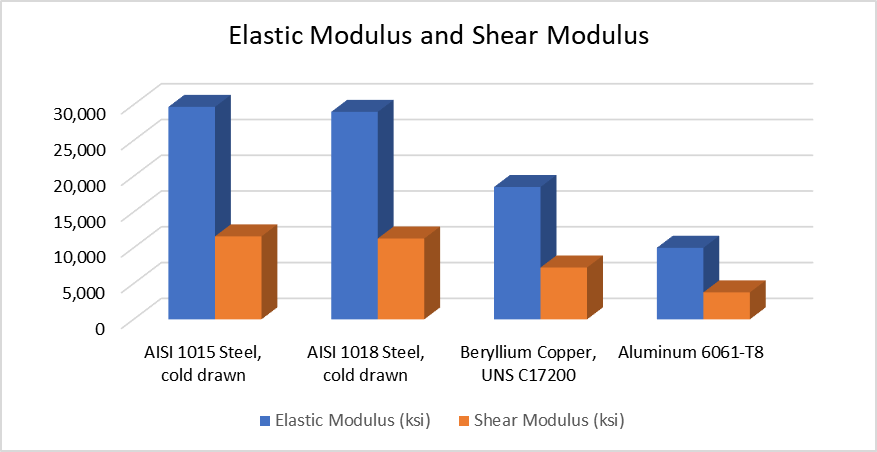# Shop Talk Blog

The Official Blog for Texas Final Drive

Mechanical stress is behind any of the failures we encounter when inspecting a final drive motor. Sheared fasteners, bent gear teeth, dented roller bearings, and broken races are examples of where stresses have gotten high enough to do permanent damage. To help you learn more about the mechanisms behind this and other types of failure, we'd like to introduce you to a new blog series entitled Dr. Mac's Notebook.

##Here are some other Shop Talk Blog posts you might find of interest:

## Simple Forces

There are three basic types of forces:

• Compressive, which pushes things together
• Tensile, which pulls things apart
• Shear, which causes things to slide apart## Mechanical Stress

Stress is a way of representing the intensity of a force and is officially defined as Force / Area.  The basic types of stress are

• Compressive
• Tensile
• Shear

There are also bending stresses, which are a combination of compressive, tensile, and shear stresses. A good example of bending stresses would be a shelf with boxes on it.

The most basic formula for stress is σ=F/A for compressive and tensile stresses and τ=V\A for shear stress. F stands for force, V stands for shear force, and A stands for the area over which the force is applied.This example applies a tensile force F = 1,000 lbs to a 1” diameter metal rod. The metal rod is welded to another surface to ensure that the rod can’t move. The cross-sectional area of the rod, over which the force is applied, comes out to 0.7854 sq. in. The resulting tensile stress on the rod is 1,274 lbs per square inch.

## Modulus of Elasticity

The modulus of elasticity measures how much deformation takes place for a certain amount of stress or stress/strain. Young’s modulus, also known as the elastic modulus, applies to tensile and elastic stresses. The greater the modulus, the less likely the material will change length at a given loading. The elastic modulus can also be considered a material's springiness.

The higher the elastic modulus, the stiffer the material, and the less it will deform under a given load. Materials with a lower elastic modulus are more likely to stretch at a given load. As the name implies, there is also a shear modulus that establishes the relationship between shear stress and shear strain.As you can see in the chart above, AISI 1015 Steel is much stiffer than either Beryllium Copper or Aluminum 6061-T8. If a tensile load was applied to a rod made from AISI 1018, it wouldn’t stretch as much as the same load applied to a rod of the same size made from Aluminum 6061-T8.

## Conclusion

Mechanical stress is at the root of many final drive failures, and recognizing the root cause can be very helpful in preventing that kind of failure again. In this blog post, we've provided a quick introduction to the basics of mechanical stress. Now, if you're having trouble with a final drive motor, give us a call at (281) 968-4473 to for some advice or to get a quote on a new or reman final drive motor.Topics: Final Drive Failure